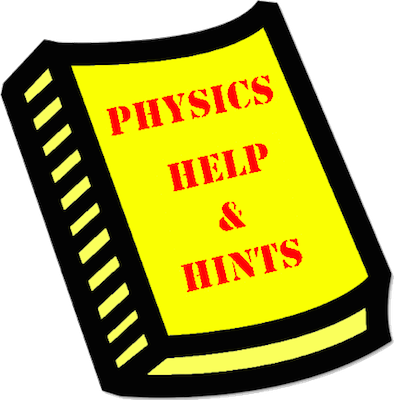# Mission SE9: Coulomb's Law Calculations

Mission SE9 pertains to the use of the Coulomb's Law equation to solve Physics word problems for F, Q, or d. The mission consists of 12 questions organized into 4 Question Groups. You must answer one question from each Question Group to complete the mission. The learning outcomes for this mission are ...

#### Learning Outcomes

• The student should be able to use Coulomb's law to calculate the electric force between two objects if given their charges and distance of separation.
• The student should be able to algebraically manipulate the Coulomb's law equation in order to determine the distance of separation between two objects if given the electric force and their charges.

### Launch Mission SE9

#### Getting Help

If you are not familiar with this topic, then you should first learn about the topic using our written Tutorial or our Video Tutorial:

The Physics Classroom - Static Electricity Unit, Lesson 3, Part b

Coulomb's Law

####Question-Specific Help

Each Question Group has its own Help page with information specific to the question. You can access the Help page from within the mission by tapping on the Help Me! icon (textbook). For your convenience, links to those pages are provided below: